# Operator Precedence and Expressions in C++

## Operator Precedence and Expressions in C++:

In this article, I am going to discuss Operator Precedence and Expressions in C++ with Examples. Please read our previous article where we discussed Arithmetic Operations in C++ with Examples. At the end of this article, you will learn everything about C++ Operator Precedence and Expressions with Examples.

##### Expressions in C++

Before explaining Precedence, let us understand the expression. The expression is just a combination of variables along with operators.

Example: a+b; here a+b is an expression. It is an algebraic expression. Expressions are nothing but formulas. The formulas that we learn in mathematics, how to write them in the program? After knowing data types and the variables, this is the very first thing that you should learn.

You should be able to perfectly write down the formula so that it gets correct results. For writing any formula in the form of expression in our program. What are the things that you should know? Let us learn.

##### Operator Precedence in C++:

We have already studied arithmetic operators in our previous article. So those arithmetic operators are addition, subtraction, multiplication, division, and mod. Along with that, there is something called precedence of the operator. Precedence is nothing but the priority of evaluating the expression when two or more operators are there.

Now let us see the precedence of arithmetic operators. Here currently we are discussing only the precedence of arithmetic operators and relational, logical, and bitwise operators also have precedence that will be discussed later.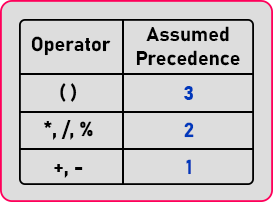So, these numbers show that parenthesis has the highest precedence and addition/subtraction is having lower precedence among arithmetic operators. We also came to know addition and subtraction as the same precedence and multiplication division and modulus as the same precedence.

##### How do these precedences affect?

Let us understand how these precedences affect. We will simply write on one formula. This is the formula we have written.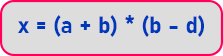Now in this formula which operator gets executed first? This actually depends on the precedence of the operator. So how many operations we have used here? Addition, multiplication, subtraction, and parenthesis.

Now, who is having higher precedence? Parenthesis has higher precedence. Then the expression under the two parentheses will be calculated first. After that, the result of the 1st parenthesis expression will be multiplied with the result of the 2nd parenthesis expression. So, this is how they are going to be executed.

##### What happens if the expression contains both multiplication and division?

If we have any expression which contains both multiplication and division then which will be executed first? In this case, the execution will start from left to right when we have the same precedence operators. This left to right is actually called associativity. So, this is how depending on the precedence the operations are performed.

##### Operator Associativity in C++:

To explain this concept, I will directly go for example. Let’s say I have an expression a*b/c where a=8, b=9, and c=10. Can you tell me the output? Since division and multiplication have the same precedence there could be two outputs.

1. 8*9/10= 7 //here 8*9 multiplied first then division happens
2. 8*9/10= 0 if the result is an integer. Here division then multiplication.

To solve this conflict operator associativity comes into the picture. This tells the compiler from where operators’ evaluation needs to be done whether it is from the left or from the right based on operator associativity. In our example, multiplication and division are left-to-right associativities. So that output will be 7.

```#include <iostream>
using namespace std;
int main ()
{
int a = 8, b = 9, c = 10;
cout << "result:" << a * b / c << endl;
return 0;
}
```

Output: result:7

For a better understanding of operator associativity, please have a look at the below table.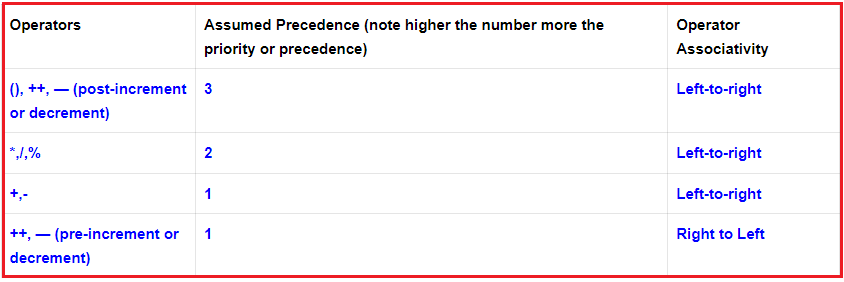##### Examples:

Next, suppose you we want any expression to execute first before any other expression then if we have,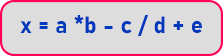In the above expression, before execution of a * b, we want b – c first. So, we will write this expression as: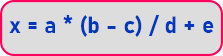Here we have added a parenthesis to execute the expression (b – c). So, in this whole expression, the parenthesis part will be executed first then multiplication or division after that addition or subtraction will be executed. The parenthesis is having the highest precedence. So, whatever it is there in the bracket that will get executed first.

Now for learning this one or for practicing this one. We have taken some formulas. We will show how to convert these formulas into expressions of the C++ program.

##### 1st formula is: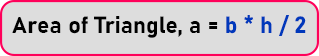The first formula is to calculate the area of a triangle.

Expression in C++ is: a = (b * h) / 2; or a = 0.5 * b * h;

We cannot write like this.

a = 1/2 * b * h;

Because we know the result of 1/2 will be zero as they are integer. So, the value of the whole expression will be zero. Here we can use typecast also. So, we can typecast it into float like:

a = (float) 1/2 * b * h;

But remember as this is written float then the result will be float. If you want you can enclose b * h in parenthesis otherwise there is no need. One thing is that you must have these variables in your program a, b and h. Otherwise, compiler gives an error.

##### 2nd formula:

Now let us move on to the next formula.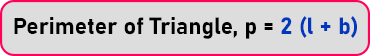The next formula is to calculate the perimeter of a rectangle.

Expression in C++ is: p = 2 * (l + b)

Here we have to use parenthesis otherwise 2 will be multiplied to l then the result will be added with b. So wrong formula will be evaluated.

##### 3rd formula:

Now the next formula is the Sum of n terms:Expression in C++ is: s = n * (n + 1) / 2

Again, we have to use parenthesis otherwise the result will be wrong.

##### 4th formula:

Now the next formula is the nth term of the A.P series that is for arithmetic progression series: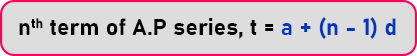Where a is the first term of the sequence, n is total terms, and d is the common difference.

Expression in C++ is: t = a + (n – 1) * d;

##### 5th formula:

The next formula is the root of the quadratic equation: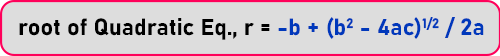This is little lengthy formula. Let us write it. r = ( -b + sqrt (b*b – 4*a*c) ) / 2*a;

Here we have used the sqrt() function of C++. It is defined in math.h header file. It is used to calculate the square root of any number. Here we calculated b2 as b*b. And for the square root part, we have used the sqrt () function of math.h library.

This was there in C language so you can use the C things in C++ also. So that header file that library is having all the mathematical functions like square root, log exponent, cos, sin, rsin, rcos, rtan, etc. You can use those mathematical functions in your program.

Now knowing about those functions slowly you can learn about them. At once you don’t have to learn all of them. So slowly as when we are using, we’ll be learning about them. But right now, we want just a square root, so there is a function called sqrt.

We have to divide the whole expression by 2a but there is an addition between the expression so we have to use parenthesis to perform addition first then divide by 2a.

##### 6th formula:

The next formula is the speed equation: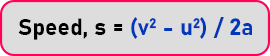Where v = final speed, u = initial speed, and a = acceleration.

Expression in C++ is: s = (v*v – u*u) / 2*a

or we can write it as: s = (pow (v, 2) – pow (u, 2)) / 2*a

Here we have used math.h library function which is pow (), It takes two parameters, that is the number and the power which we want to calculate. Now we have done enough examples on how to write expressions, now the same expressions we will use in the program.

##### FAQs:
###### What is sqrt()?

For using sqrt() you have to include a header file using any one method.
1. #include<cmath>
2. #include<math.h>

###### What is the data type of expression?

The data type of an expression will be the same as the largest data type used in the expression. For example:
int x=10;
float y=3;
float z=x/y; the result will be in float because y is float.

###### What is Typecasting?

If you want to change the data type of the result of expression using typecasting. For example:
int x=10, y=3;
float z;
z=x/y; // both x and y are int type so the result will also be int. So, z=3. Even though z is float.
z=(float)x/y; //result will be obtained in float so z=3.333.

###### Left and Right side of =

The data types of the left- and right-hand sides of expression are not related. For Example:
int x=10, y=3; // here both x and y are integer type
float z=x/y; // here z is float but the result of x/y will be double. Thought z is float.
float z=(float)x/y; // you have to typecast to get the result in float.

##### Assignment for you:

Please try to solve the below Assignments on Arithmetic Operators, Precedence, and Associativity. The solution will be given in this article. please see it in case you were stuck.

1. Program to find the area of a rectangle.
2. Program to calculate the Simple interest.

In the next article, I am going to discuss Program Using Expression in C++ with Examples. Here, in this article, I try to explain Operator Precedence and Expressions in C++ with Examples and I hope you enjoy this Operator Precedence and Expressions in C++ article.